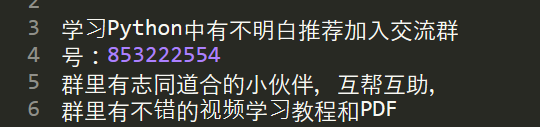↓↓↓↓↓↓1024程序员节，京东图书满100减50！ ↓↓↓↓↓↓↑↑↑↑↑↑1024程序员节，京东图书满100减50！ ↑↑↑↑↑↑

Python 里有个小彩蛋：for i in range(len(lst)): print(lst[i])

for i in lst: print(i)

1、交换两个变量的值，普通写法：

temp = aa = bb = temp

pythonic 写法：

a, b = b, a

2、类似的解包（unpacking）用法还可以实现多个返回值的函数。普通写法：

def func(a, b): result = [b, a]

return resultr = func(a, b)x = ry = r

pythonic 写法：

def func(a, b): return b, ax, y = func(a, b)

3、读写文件，普通写法：

f = open('filename.txt')text = f.read()print(text)f.close()

pythonic 写法：

with open('filename.txt') as f:

for line in f: print(line)

with 的好处是即使出错，也会帮你关闭文件。

4、拼接字符串，普通写法：

letters = ['h', 'e', 'l', 'l', 'o']s = ''for l in letters: s += lprint(s)

pythonic 写法：

print(''.join(letters))

5、关于前面遍历列表的例子，如果你想带上索引，可以这么写：

for i, elem in enumerate(lst): print(i, elem)

for key, value in dct.items(): print(key, value)

6、取出列表中大于 0 的元素，生成新列表。普通写法：

new_lst = []

for i in lst:

if i > 0: new_lst.append(i)

pythonic 写法：

new_lst = [i for i in lst if i > 0]

new_lst = (i for i in lst if i > 0)

for i in new_lst: print(i)

7、判断一个值是否为True、是否为空列表、是否是None，普通写法：

if x == True:

pass

if len(y) == 0:

pass

if z == None:

pass

pythonic 写法：

if x:

pass

if not y:

pass

if z is None:

pass

8、根据键名获取字典中对应的值，普通写法：

value = dct[key]

pythonic 写法：

value = dct.get(key, 0)

1. 多看。看官方库、优秀项目，学习别人的代码。以及看一些优质的教程和经验分享，
2. 多搜。当你实现一个小功能后，去网上搜一下，别人是怎么写的，对比下是不是比你自己的更好。举个例子：如何从列表中删除重复元素。你自己可以通过循环实现，但只要搜一下，就会知道 list(set(x)) 这种用法。
3. 多写。自己还没写几行代码，就先别纠结什么效率什么风格了。最终还是要写上足够量的代码，才会有“悟道”的那一刻。